#### FieldStrength

##### Description

FieldStrength[μ, ν, a] is the field strength tensor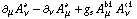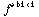. FieldStrength[μ, ν] is the field strength tensor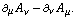The name of the field (A) and the coupling constant (g) can be set through the options or by additional arguments. The first two indices are interpreted as type LorentzIndex, except OPEDelta, which is converted to Momentum[OPEDelta].

``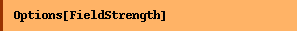``
``

##### Examples
``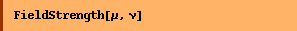``
`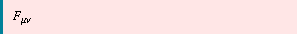`
``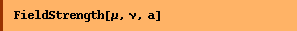``
`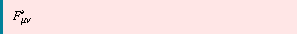`
``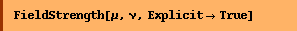``
`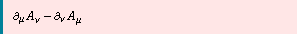`
``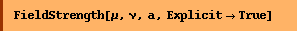``
`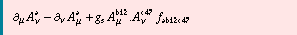`
``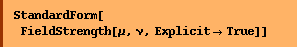``
`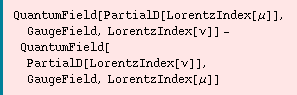`
``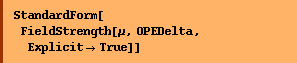``
`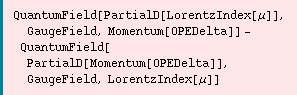`
``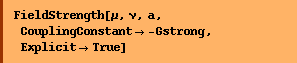``
`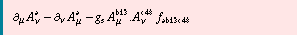`

Converted from the Mathematica notebook FieldStrength.nb